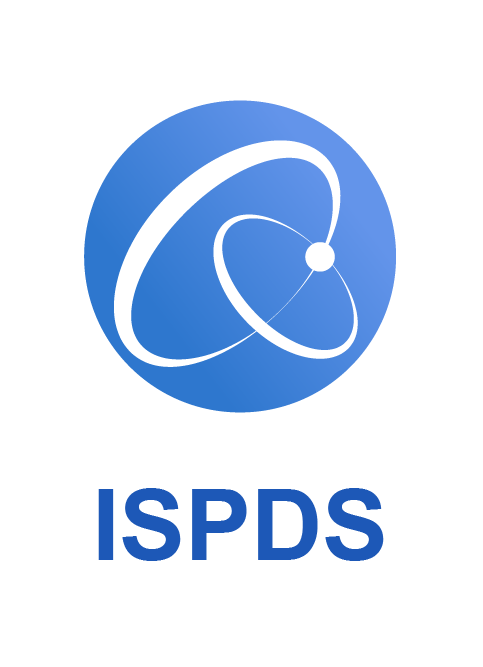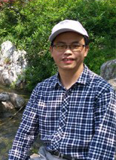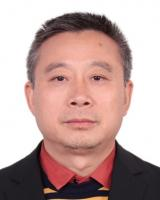2nd International Conference on Information Science, Parallel and Distributed Systems
Countdown
Speaker

Keynote speaker IProf. Zhu Quanxin

Distinguished Professor and Doctoral Supervisor, Hunan Normal University, China

Topic: Stabilization of stochastic nonlinear delay systems via the event-triggered feedback control

Abstract: In practical systems, it often happens that some stochastic differential equations (SDEs) are unstable. It is an interesting and challenging question: How to design the control to ensure the stability of SDEs? Different from the traditional works, we use the event-triggered feedback control to solve this problem. Compared with large number of results for discrete-time stochastic systems, only a few results have appeared on the event-triggered control for continuous-time stochastic systems, but the event-triggered control for continuous-time stochastic delay systems is not unsolved. In this talk, we will give a positive answer to this question.

Keynote speaker IIProf. Daowen Qiu

School of Data and Computer Science, Sun yat-sen University

Topic: Quantum exact 1-query complexity

Abstract: Quantum query algorithms are very important problems in quantum computing, for example, Deutsch-Jozsa algorithm, Simon's algorithm, Shor's algorithm, Grover's  algorithm, and HHL algorithm belong to quantum query algorithms. Quantum exact query algorithms mean obtaining the results without error, such as Deutsch-Jozsa algorithm, Simon's algorithm, Grover's algorithm. These algorithms confirm the advantages of quantum computing over classical computing, and are pioneering achievements of quantum computing. In this talk, I would like to present from three aspects:

(I)  We first would like to recall the research background and new progresses of quantum query algorithms, especially focusing on quantum exact query algorithms.

(II)  Then we would introduce the main results we have obtained in recent years: (1) we have discovered all partial symmetric Boolean functions with quantum exact 1-query complexity, and these functions can be computing exactly by Deutsch-Jozsa algorithm. A direct corollary is that we have obtained all total Boolean functions with quantum exact 1-query complexity. (2) we have obtained a sufficient and necessary condition for partial Boolean functions with quantum exact 1-query complexity, and then all total Boolean functions with quantum exact 1-query complexity directly follow, as well. (3) we have constructed a concrete partial Boolean function with quantum exact 1-query complexity and proved this function cannot be computed by Deutsch-Jozsa algorithm.

(III)  Finally, we would like to point out a number of problems for further study.

Keynote speaker IIIProf. Ouyang Jianquan

Xiangtan University, China

Research Area:

Multi-modal Mashup, Multimedia Analysis and Retrieval, Multi-valued Logic, Trusted Computing, Deep Learning, MRC Image Processing, Cyberspace Security